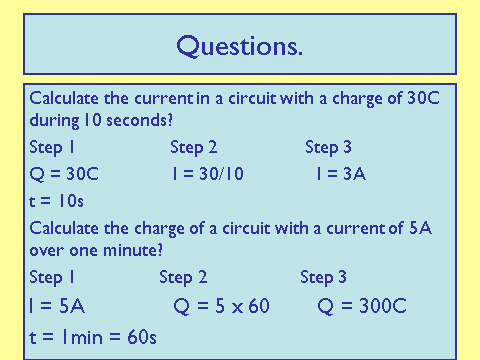# Current

## Ammeter

An ammeter is used to measure current, measured in Amps, A. It is always placed in series.

## Current

Current is the rate of flow of charge and in metals it is the flow of electrons.

﻿﻿

## Equation

You must be able to remember and apply this equation: charge flow = current x time

This can also be written as: Q = It

• Q = Charge (Coulombs, C)
• I = Current (Amps, A)
• t = Time (Seconds, s)

Around a single loop the current always remains the same.

Let’s look at some examples:Notice, in the second example, time is changed from minutes to seconds. Always use seconds!! Never minutes or hours.

Also, look at the three steps in showing ones working. You should always show your working, even if you use a calculator - and never forget to include units!

What is the unit of Charge?
Your answer should include: Coulomb / Coulombs / C
Calculate the charge which passes a point in a circuit with a current of 10A in 45 seconds.
450C
Calculate the current with 40kC of charge passing a point in 5ms. Hint: 1kC = 1000C 1millisecond = 0.001 second
Your answer should include: 8 x 10^6 A / 8000000 A